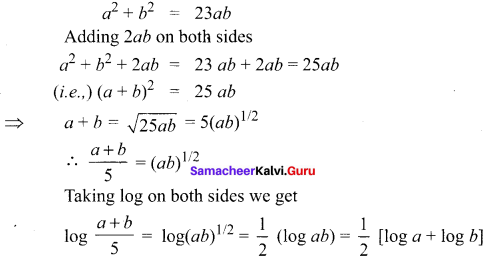You can Download Samacheer Kalvi 11th Maths Book Solutions Guide Pdf, Tamilnadu State Board help you to revise the complete Syllabus and score more marks in your examinations.

## Tamilnadu Samacheer Kalvi 11th Maths Solutions Chapter 2 Basic Algebra Ex 2.12

Question 1.
Let b > 0 and b ≠ 1. Express y = bx in logarithmic form. Also state the domain and range of the logarithmic function.
Solution:
Given y = bx ⇒ logby = x, x ∈ R with range (0, ∞) (-∞, ∞)

Question 2.
Compute log927 – log279.
Solution:
Let log927 = x ⇒ 27 = 9x ⇒ 33 = (32)x = 32x
⇒ 2x = 3 ⇒ x = 3/2
Let log279 = x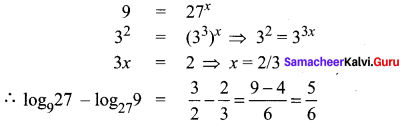Question 3.
Solve log8x + log4x + log2x = 11
Solution: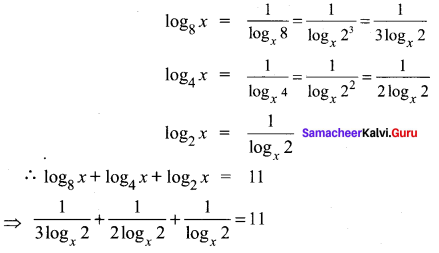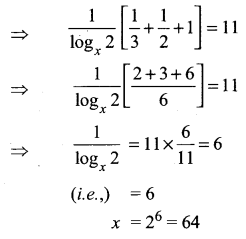Question 4.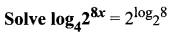Solution: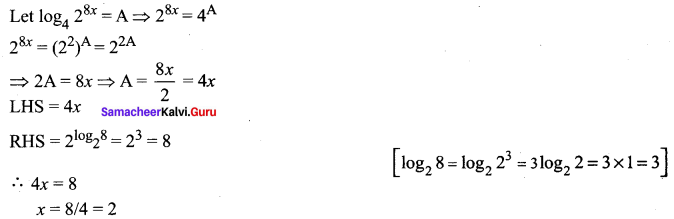Question 5.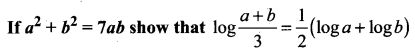Solution: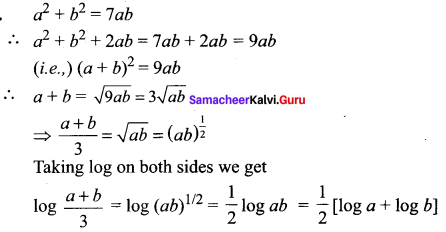Question 6.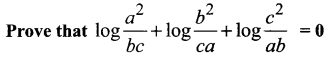Solution: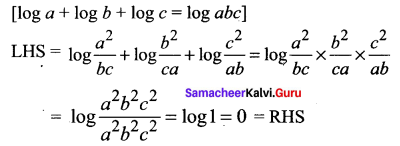Another methods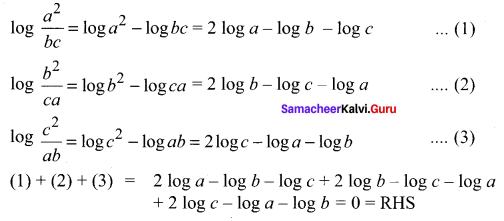Question 7.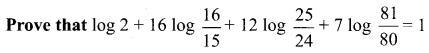Solution: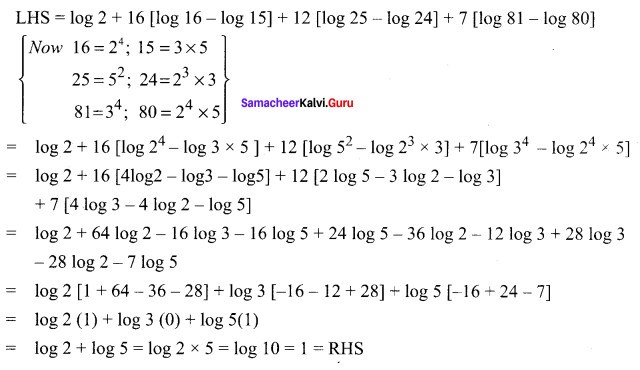Question 8.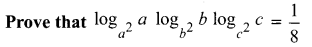Solution: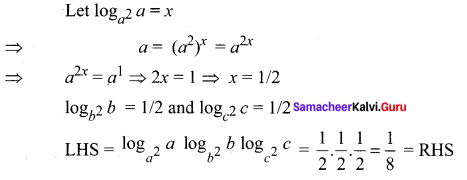Question 9.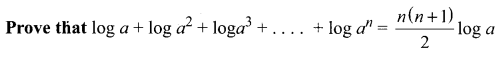Solution: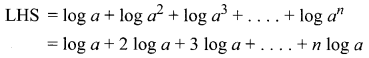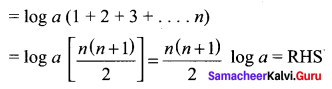Question 10.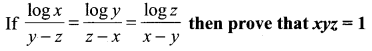Solution: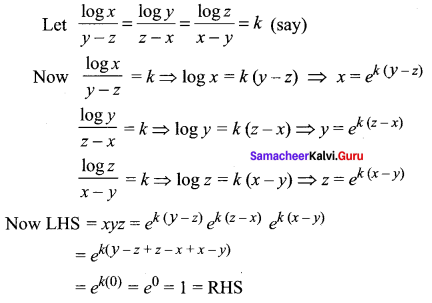Question 11.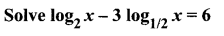Solution: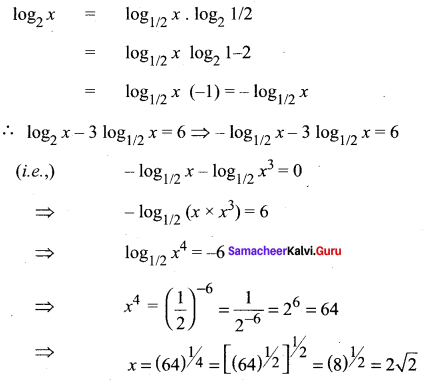Question 12.
Solve log5 – x(x2 – 6x + 65) = 2
Solution:
log5 – x(x2 – 6x + 65) = 2
⇒ x2 – 6x + 65 = (5 – x)2
x2 – 6x + 65 = 25 + x2 – 10x
⇒ x2 – 6x + 65 – 25 – x2 + 10x = 0
4x + 40 = 0 ⇒ 4x = -40
x = -10

### Samacheer Kalvi 11th Maths Solutions Chapter 2 Basic Algebra Ex 2.12 Additional Questions

Question 1.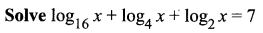Solution: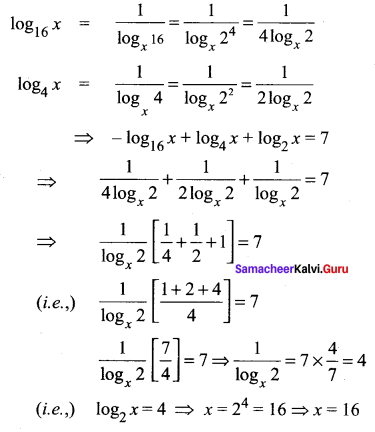Question 2.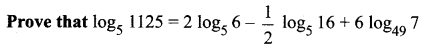Solution: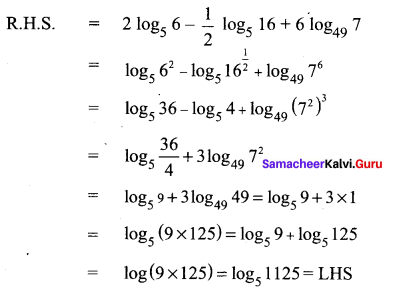Question 3.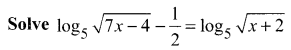Solution: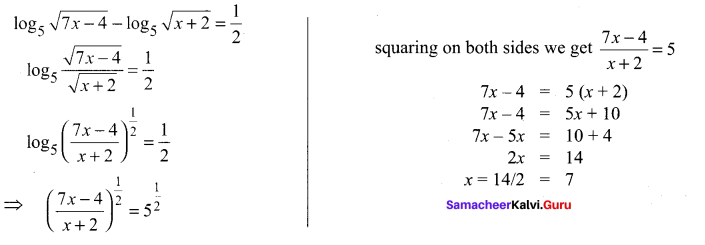Question 4.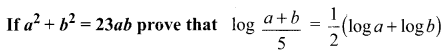Solution: